• 08258-262724
• principalaiet08@gmail.com
• CET CODE-169

## Alva's Institute of Engineering & Technology

#### A Unit of Alva's Education Foundation(R), Moodubidire

(Affilliated to VTU, Belgaum, Approved by AICTE, New Delhi, Recognized by Govt. of Karnataka)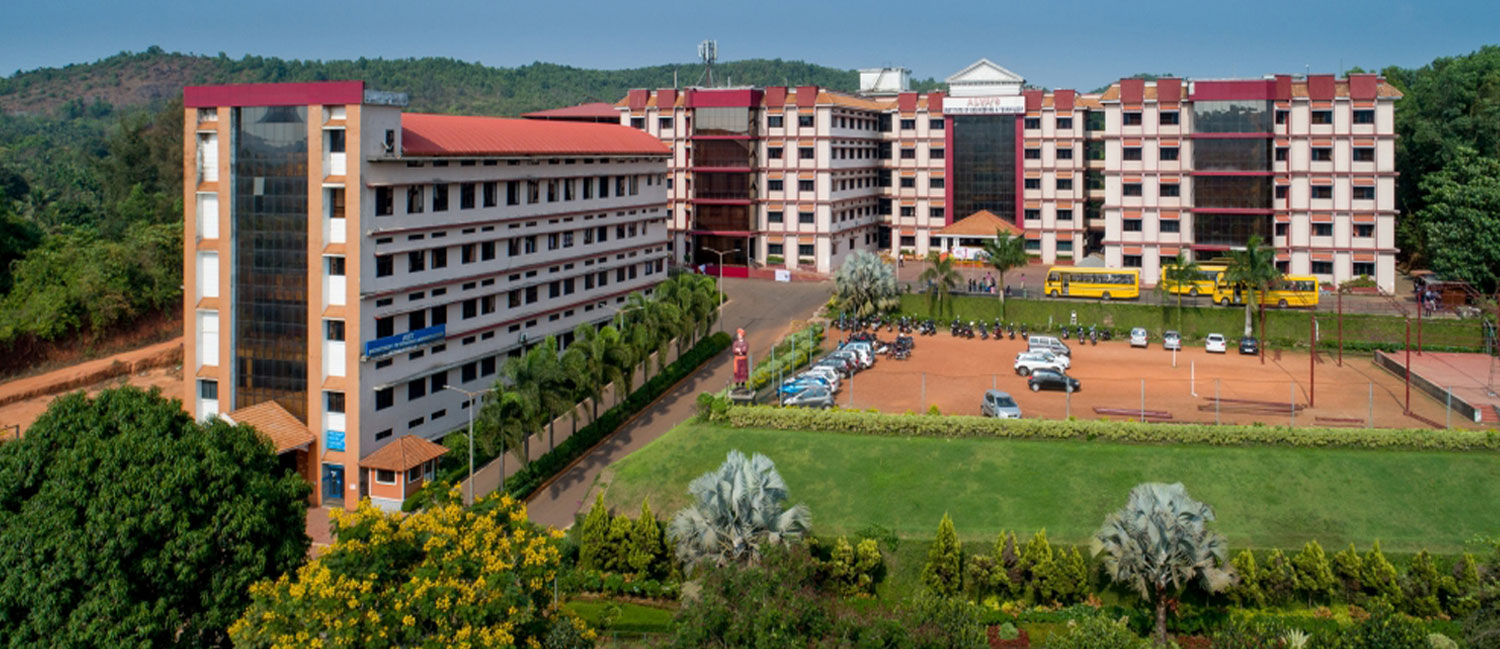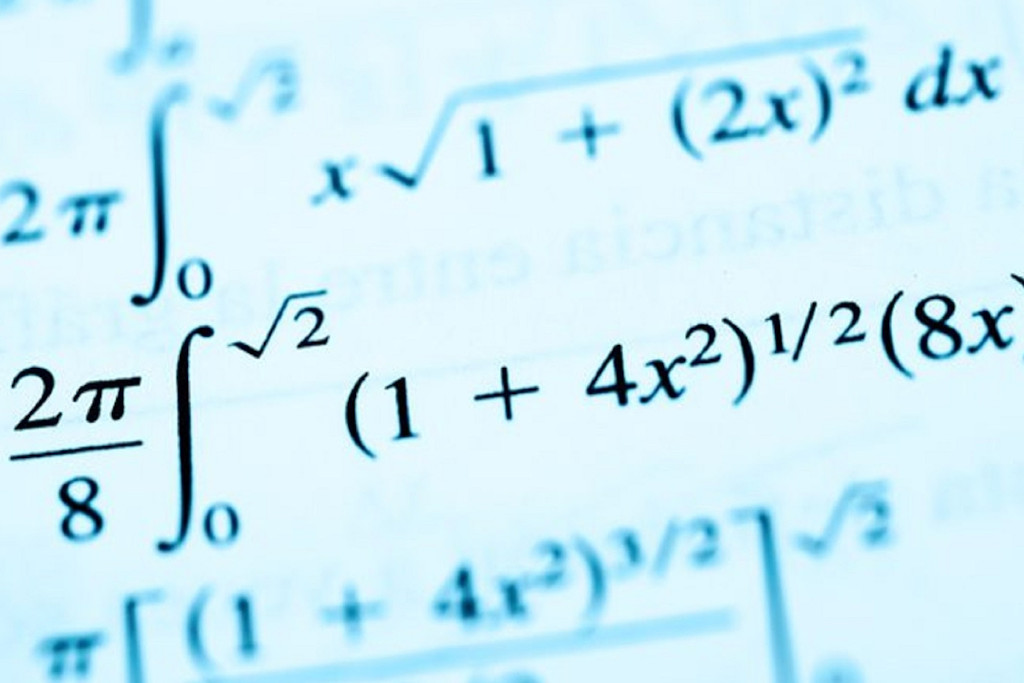# Mathematics

Department of Mathematics came into existence from the inception of the Institution in the year 2008. The department is supporting technical branches by teaching students the basics of mathematics, as per the curriculum. The department is consisting of dedicated staff members, who are well qualified, experienced in the field of teaching, who are capable of meeting the needs of students. Along with teaching, the department is extending support to research-oriented minds, by providing the opportunity to do research in the VTU recognised research centre of Mathematics.

16 %

9 +

+

4 +
###### Research Grant in Lakhs
• Vision

“To develop quality engineers by creating enthusiasm towards Mathematics and its applications through the depth of its teaching and research”.

• Mission
• Making Engineers to develop reasoning ability, analytical skills to solve complex engineering problems.
• Creating the ability to design mathematical modelling through the applications of mathematics for systems involving real-life problems.
• Providing new knowledge by triggering creativity in students through research programmes.
• COURSE OUTCOMES(COs)

EVEN:

Mathematics-II for Civil Engineering stream (BMATC201)

• Apply the knowledge of multiple integrals to compute area and volume.
• Understand the applications of vector calculus refer to solenoidal, irrotational vectors, line integral and surface integral.
• Demonstrate partial differential equations and their solutions for physical interpretations.
• Apply the knowledge numerical methods in solving physical and engineering phenomena.
• Get familiarize with modern mathematical tools namely SCILAB/PYTHON/MATLAB.

Mathematics-II for Mechanical Engineering stream (BMATM201)

• Apply the knowledge of multiple integrals to compute area and volume.
• Understand the applications of vector calculus refer to solenoidal, irrotational vectors, line integral and surface integral.
• Demonstrate partial differential equations and their solutions for physical interpretations.
• Apply the knowledge numerical methods in solving physical and engineering phenomena.
• Get familiarize with modern mathematical tools namely SCILAB/PYTHON/MATLAB

Mathematics-II for Electrical & Electronics Engineering Stream (BMATE201)

• Understand the applications of vector calculus refer to solenoidal, irrotational vectors, line integral and surface integral.
• Demonstrate the idea of Linear dependence and independence of sets in the vector space, and linear transformation.
• To understand the concept of Laplace transform and to solve initial value problems.
• Apply the knowledge numerical methods in solving physical and engineering phenomena.
• Get familiarize with modern mathematical tools namely SCILAB/PYTHON/MATLAB.

Mathematics-II for Computer Science and Engineering stream (BMATS201)

• Apply the concept of change of order of integration and variables to evaluate multiple integral and their usage in computing area and volume.
• Understand the applications of vector calculus refer to solenoidal, irrotational vectors, orthogonal curvilinear coordinates.
• Demonstrate the idea of Linear dependence and independence of sets in the vector space,and linear transformation.
• Apply the knowledge of numerical methods in analysing the discrete data and for solving the physical and engineering problems.
• Get familiarize with modern mathematical tools namely SCILAB/PYTHON/MATLAB.

COMPLEX ANALYSIS, PROBABILITY AND STATISTICAL METHOD (21MAT41)

• Use the concepts of analytic function and complex potentials to solve the problems arising in electromagnetic field theory. Utilize conformal transformation and complex integral arising in aerofoil theory, fluid flow visualization and image processing.
• Obtain series solutions of Ordinary Differential Equation.
• Make use of the correlation and regression analysis to fit a suitable mathematical model for the statistical data.
• Apply discrete and continuous probability distributions in analyzing the probability models arising in engineering field.
• Construct joint probability distributions and demonstrate the validity of testing the hypothesis.

MATHEMATICAL FOUNDATIONS FOR COMPUTING, PROBABILITY AND STATISTICS (21MATCS41)

• Apply the concepts of logic for effective computation and relating  problems in the Engineering domain.
• Analyse the concepts of functions and relations to various fields of Engineering. Comprehend the concepts of Graph Theory for various applications of computational sciences.
• Make use of the correlation and regression analysis to fit a suitable mathematical model for the statistical data.
• Apply discrete and continuous probability distributions in analyzing the probability models arising in engineering field.
• Construct joint probability distributions and demonstrate the validity of testing the hypothesis.

COMPLEX ANALYSIS, PROBABILITY AND LINEAR PROGRAMMING (21MATME41)

• Use the concepts of analytic function and complex potentials to solve the problems arising in fluid flow.
• Utilize conformal transformation and complex integral arising in aerofoil theory, fluid flow visualization and image processing.
• Apply discrete and continuous probability distributions in analyzing the probability models arising in engineering field.
• Analyse and solve linear programming models of real-life situations and solve LPP by the simplex method.
• Learn techniques to solve Transportation and Assignment problems.

ODD:

Mathematics-I for Civil Engineering stream (BMATC101)

• Apply the knowledge of calculus to solve problems related to polar curves and learn the notion of partial differentiation to compute rate of change of multivariate functions.
• Analyze the solution of linear and nonlinear ordinary differential equations.
• Get acquainted and to apply modular arithmetic to computer algorithms.
• Make use of matrix theory for solving the system of linear equations and compute eigenvalues and eigenvectors.
• Familiarize with modern mathematical tools namely MATHEMATICA/MATLAB/ PYTHON/ SCILAB.

Mathematics-I for Mechanical Engineering stream (BMATM101)

• Apply the knowledge of calculus to solve problems related to polar curves.
• Learn the notion of partial differentiation to compute rate of change of multivariate functions.
• Analyze the solution of linear and nonlinear ordinary differential equations.
• Make use of matrix theory for solving the system of linear equations and compute eigenvalues and eigenvectors.
• Familiarize with modern mathematical tools namely MATHEMATICA/MATLAB/ PYTHON/ SCILAB.

Mathematics-I for Electrical & Electronics Engineering Stream (BMATE101)

• Apply the knowledge of calculus to solve problems related to polar curves and learn the notion of partial differentiation to compute rate of change of multivariate functions.
• Analyze the solution of linear and nonlinear ordinary differential equations.
• Apply the concept of change of order of integration and variables to evaluate multiple integrals and their usage in computing area and volume.
• Make use of matrix theory for solving the system of linear equations and compute eigenvalues and eigenvectors.
• Familiarize with modern mathematical tools namely MATHEMATICA/MATLAB/ PYTHON/ SCILAB.

Mathematics-I for Computer Science and Engineering stream (BMATS101)

• Apply the knowledge of calculus to solve problems related to polar curves and learn the notion of partial differentiation to compute rate of change of multivariate functions.
• Analyze the solution of linear and nonlinear ordinary differential equations.
• Get acquainted and to apply modular arithmetic to computer algorithms.
• Make use of matrix theory for solving the system of linear equations and compute eigenvalues and eigenvectors.
• Familiarize with modern mathematical tools namely  MATHEMATICA/MATLAB/ PYTHON/ SCILAB.

Transform Calculus, Fourier series and Numerical Techniques (21MAT31)

• To solve ordinary differential equations using Laplace transform.
• Demonstrate Fourier series to study the behaviour of periodic functions and their applications in system communications, digital signal processing and field theory.
• To use Fourier transforms to analyze problems involving continuous-time signals and to apply Z-Transform techniques to solve difference equations.
• To solve mathematical models represented by initial or boundary value problems involving partial differential equations.
• Determine the extremals of functional using calculus of variations and solve problems arising in dynamics of rigid bodies and vibrational analysis.

CALCULUS AND DIFFERENTIAL EQUATIONS (21MAT11)

• Apply the knowledge of calculus to solve problems related to polar curve and its applications in determining the bentness of a curve.
• Learn the notion of partial differentiation to calculate rates of change of multivariate functions and solve problems related to composite functions and Jacobeans.
• Solve first order linear/nonlinear differential equation analytically using standard methods.
• Demonstrate various models through higher order differential equations and solve such linear ordinary differential equations.
• Test the consistency of a system of linear equations and to solve them by direct and iterative methods.

CALCULUS AND LINEAR ALGEBRA (18MAT11)

• Apply the knowledge of calculus to solve problems related to polar curve and its applications in determining the bentness of a curve.
• Learn the notion of partial differentiation to calculate rates of change of multivariate functions and solve problems related to composite functions and Jacobeans.
• Apply the concept of change of order of integration and variables to evaluate multiple integrals and their usage in computing the area and volumes.
• Solve first order linear/nonlinear differential equation analytically using standard methods.
• Make use of matrix theory for solving system of linear equations and compute eigen values and eigenvectors required for matrix diagonalization.

Advanced Calculus and Numerical methods (18MAT21)

• Apply multivariate calculus to understand the Solenoidal and irrotational vectors and also exhibit the inter dependence of line, surface and volume integrals.
• Construct various physical models through higher order differential equations and solve such linear ordinary differential equations.
• Construct a variety of partial differential equations and solution by exact methods/method of separation of variables.
• Explain the applications of infinite series and obtain series solution of ordinary differential equations.
Apply the knowledge of numerical methods in the modelling of various physical and engineering phenomena.

Transform Calculus, Fourier Series and Numerical Techniques (18MAT31)

• Use Laplace transform and Inverse Laplace transform in solving differential / Integral equation arising in network analysis, control systems and other fields of engineering.
• Demonstrate Fourier series to study the behaviour of periodic functions and their applications in system communications, digital signal processing and field theory.
• Make use of Fourier transform and Z-transform to illustrate discrete/ continuous function arising in wave and heat propagation, signals and systems.
• Solve first and second order ordinary differential equations arising in engineering problems using single step and multi-step numerical methods.
• Determine the extremals of functionals using calculus of variations and solve problems arising in dynamics of rigid bodies and vibrational analysis.

Complex Analysis, Probability and Statistical Methods (18MAT41)

• Use the concepts of analytic function and complex potentials to solve the problems arising in electromagnetic field theory.
• Utilize conformal transformation and complex integral arising in aerofoil theory, fluid flow visualization and image processing.
• Apply discrete and continuous probability distributions in analyzing the probability models arising in engineering field.
• Make use of the correlation and regression analysis to fit a suitable mathematical model for the statistical data.
• Construct joint probability distributions and demonstrate the validity of testing the hypothesis.

National Mathematics Day - 2022(January 20,2023)

National Mathematics Day - 2021 (December 30, 2021)

The Birth anniversary of renowned Indian Mathematician Srinivasa Ramanujan, December 22, was announced as National Mathematics Day by then Prime Minister Dr. Manmohan Singh on the 125th birthday of Srinivas Ramanujan at Madras University. Henceforth, various educational institutions throughout the nation are celebrating the day with great enthusiasm. With great spirit, the Department of Mathematics, AIET, Mijar celebrated National Mathematics Day 2021 on December 30, 2021, at the Auditorium of AIET.
On behalf of National Mathematics Day, mathematics puzzle-solving and science model competitions were held for students. The puzzle-solving competition was conducted on December 23, 2021 at 3.30 PM in Rooms No. 403 and 412 of the Main Block. 87 students from first year to final year actively participated in this competition. The Science Model Competition was held on the day of celebration, i.e., December 30, 2021.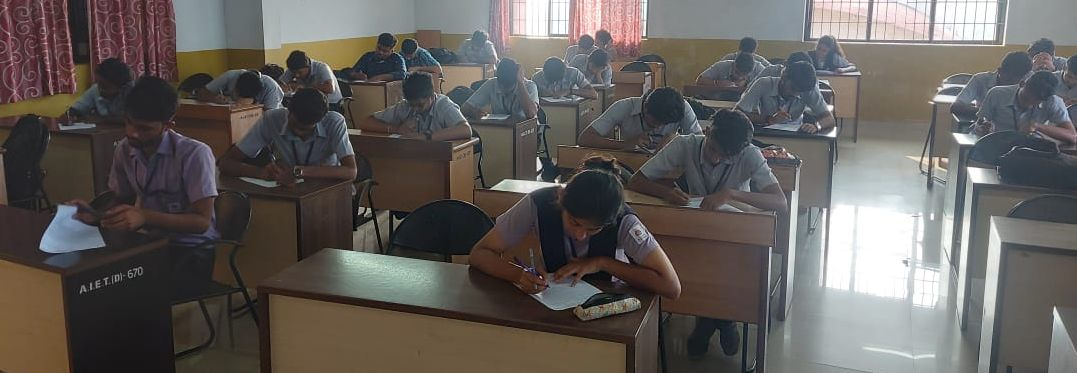Mr. Yakoob Koyyur, Head Master of GHS Nada, Belthangady, Chief guest for the programme, Dr. Shashi Kumar from the Physics Dept., and Dr. Ajith Hebbar from the Civil Engg. Dept., judged the competition. The principal, faculty, and students from various branches witnessed the program. Soon after the competition, the formal function started. Nithin Hemaraj hosted the program. The program started with a prayer by Sharanya and Arundhathi from 5th semester ISE. Then Dr. Prashanthi K. S. welcomed the dignitaries and guests and introduced the Chief Guest Mr. Yakub Koyyur. Sathyam Pawale from 3rd-semester AIML announced the prize winners’ names and the Chief Guest handed over the prize.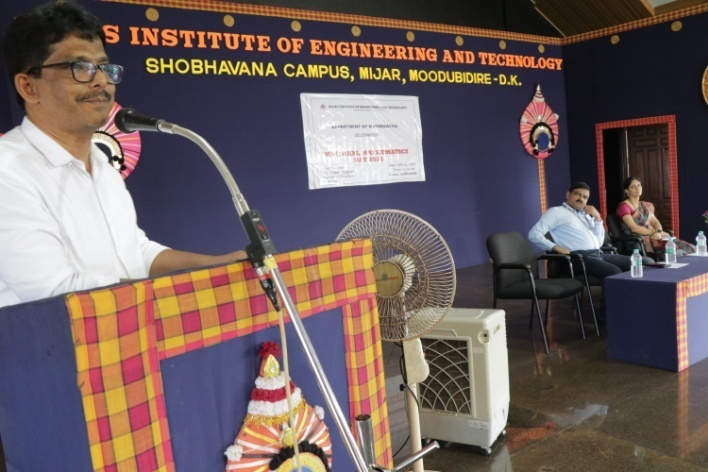The winners of the mathematics puzzle-solving competition were Nithin Hemaraj from 3rd-semester of AIML secured 1st place and 2nd place was secured by two students, Sayeed A.R. from CSE 3rd semester and Ullas H.U. from AIML 3rd semester.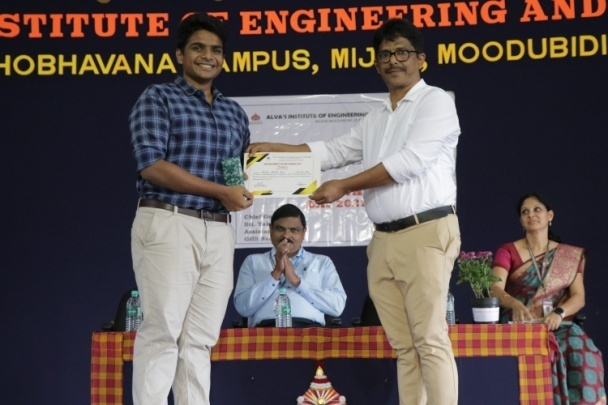K K Kaushik and team from 3rd semester CSE won first place, Satyam Pawale and team from 3rd semester AIML got 2nd place, and Fathima Thashiba and team from 3rd semester received 3rd place in the Science Model Making Competition.

Then Dr. Prameela Kolake, HoD of the Mathematics Department, gave preliminary speech, which was followed by the launch of the e-magazine “Mathroot” by the dignitaries. Mathroot contains content and concepts about mathematics for learners.

After the launch of the e-magazine, Chief Guest addressed the gathering and, in his address, Mr. Yakub Koyyur, beautifully narrated Srinivasa Ranujan’s life story and also advised the students about the importance of studies in life by giving examples of great souls like Dr. A P J Abdul Kalam, Sir M Visweshwarayya. The principal then addressed and encouraged the gathering to take mathematics not as a subject and treat it as a source of enjoyment and development. At the end, Keerthana from 3rd-semester AIML delivered a vote of thanks. As Twilight drops her curtain down and pins it with a star, the programme ended with the wonderful memories of the day.

Workshop 3: Five days National level workshop on Maxima & Sage Math (21st to 25th September 2021)
The department of Mathematics has organized five days National Level workshop on “Maxima and Sage Math” from 21st (Tuesday) to 25th (Saturday) of September, 2021 with the objective to understand the contributions of these tools in strengthening the conceptual understanding, visualizing and problem solving genuinely. All the sessions (hands-on sessions) of the workshop were organized through online mode using Cisco Webex Platform and Google meet.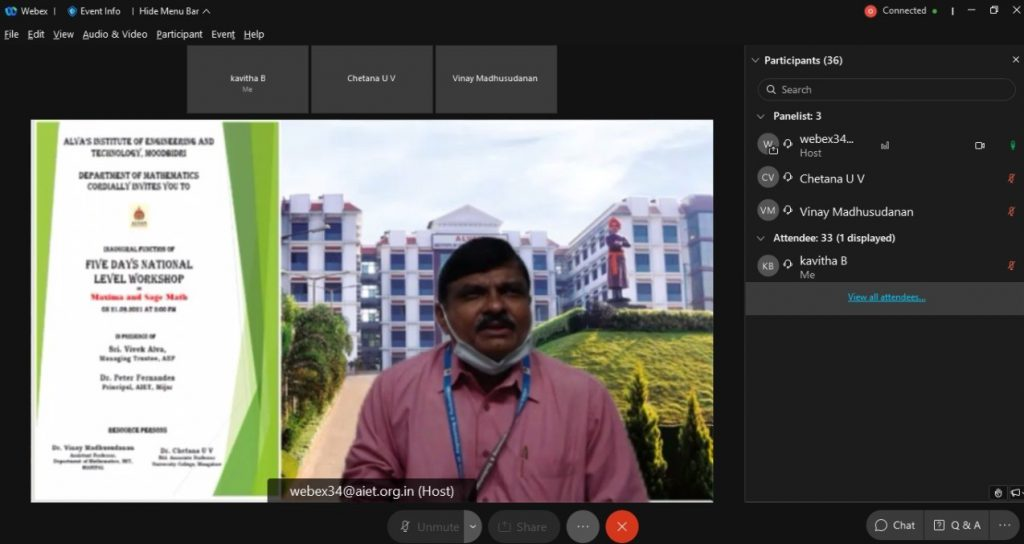Dr. Peter Fernandes., Principal, Alva’s Institute of Engineering and Technology, Mijar, was the Chief Guest for the inaugural function which held at Board room of the institution.

Resource persons Mr.Vinay Madhusudanan, Dr.Chetana U V and Heads and faculties from other departments of the institution were also present on this occasion.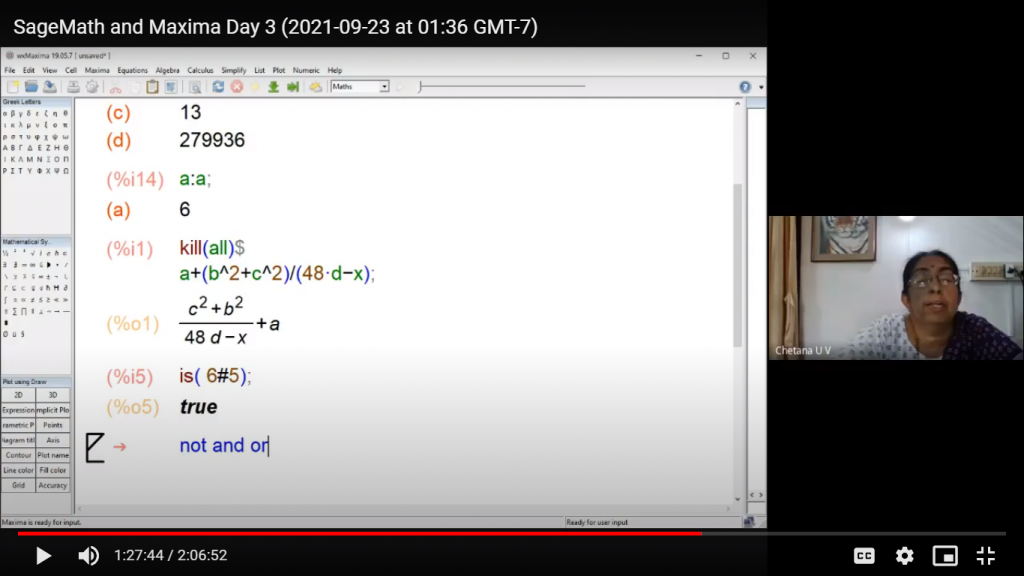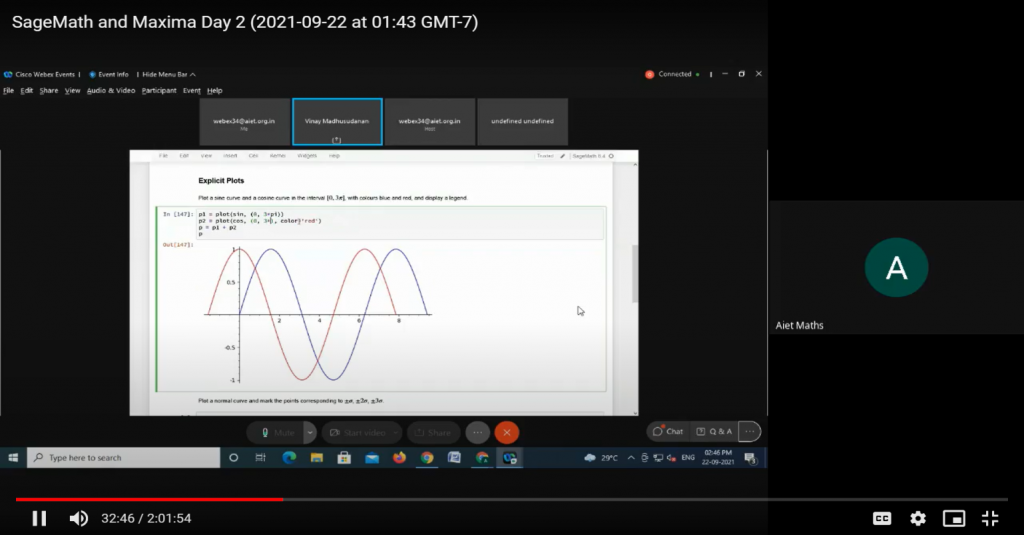25 faculty, 12 students from various colleges and 05 research Scholars from different research centers participated in this workshop.

Mr.Vinay Madhusudanan, Assistant Professor, Department of Mathematics, Manipal Institute of Technology, Manipal, was the resource person for the hands-on session on “Sage Math” that took place from September 21st to September 23rd, 2021. Dr. Chetana U V, Rtd Associate Professor, Department of Mathematics, University College, Mangalore, served as the resource person for the “Maxima” hands-on session, which took place from September 23rd to September 25th, 2021.

All of the sessions went well, and the attendees’ overall impressions of the workshop were positive.

Mathematics puzzle solving competition

The department of Basic science has organized weekend activities for first year students in the month of March, 2021. On behalf of Mathematics department, Maths Puzzle solving competition was conducted on 06.03.2021. First year students from all the sections actively participated in the event. 215 students were enrolled for Math’s Puzzle competition.

Students were asked to solve 15 puzzles involving basic mathematics knowledge. Out of 215 participants 3 students had a tie with 9 out of 15 marks namely Nitin Hemaraj, Prajwal P Pavsekar, Sayeed Abdul Rahman. A tie breaker round was conducted on 13.03.2021. In the tie breaker round Nithin Hemaraj was announced as winner and Sayeed Abdul Rahman was runner-up.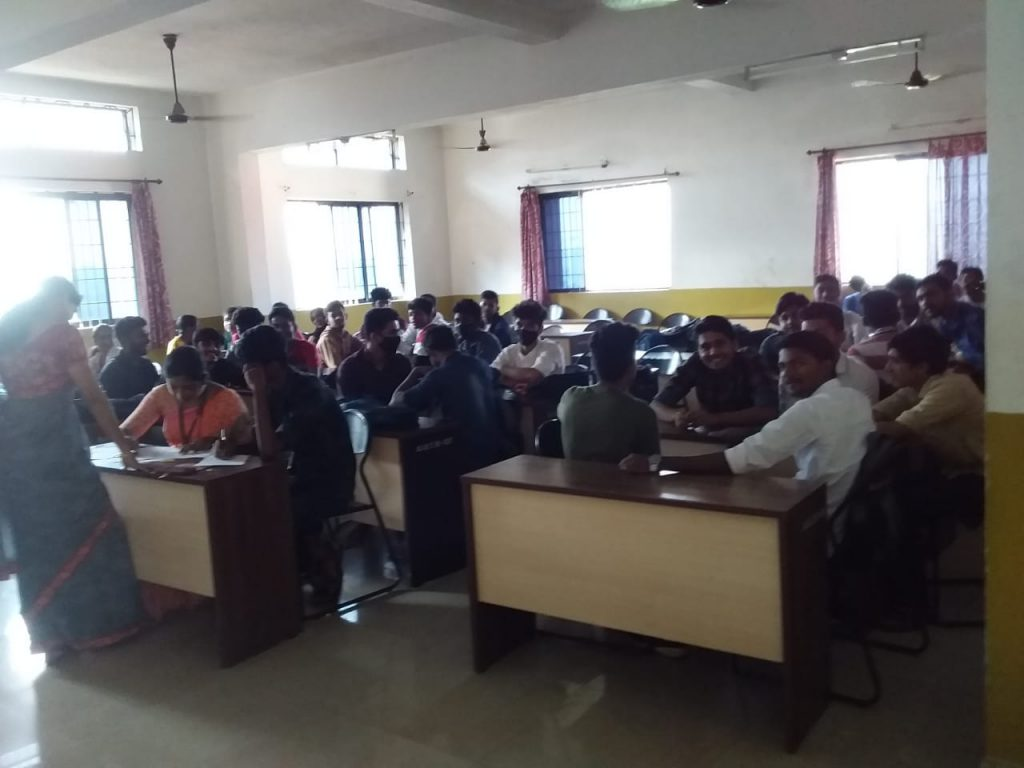Workshop 2: Two days workshop on “Application of Mathematics in science and Technology” (January 20th & 21th, 2017)
A two day workshop on “APPLICATION OF MATHEMATICS IN SCIENCE AND TECHNOLOGY” has been conducted by the department of Engineering Mathematics on 20th (Friday) and 21st (Saturday) of January, 2017. The workshop was inaugurated by Dr. Praveenraj C. Principal, Alva’s Homeopathy Medical College, Mijar and he conveyed how mathematics is applicable in the field of medicine. Academic Dean Dr.Basavaraju, Prof. Prameela Konde,HOD/ Maths department, resource person of the workshop Dr. Shyam S. Kamath, Deans and HODs of various departments, all staff members and student volunteers were also present during the inauguration function.

31 faculties from various colleges, 59 students from Alva’s college of PG studies and two students from other colleges were participated in the workshop.

Workshop 1: One day workshop on Mathematics and its applications in engineering and technology (20th JANUARY 2014)
A one day workshop on “Mathematics and its applications in Engineering and Technology” has been conducted by the department of Engineering Mathematics on 20-01-2014. The workshop was inaugurated by Dr. Narasimhamurthy, Professor & chairman, Dept. of mathematics, Kuvempu University, Shimoga and presided over by Dr. Peter Fernandes, Principal, AIET,Moodabidri. Prof. M Ramananda Kamath, HOD/ Maths Dept, Resource Person of the workshop, Dr. M Sankar, Deans and HODs of various departments, staff members and student volunteers were also present during the inauguration function. Total 59 Participants from 9 various colleges were participated.

 Sanctioned Funds Rs. Four lakhs has been sanctioned by Vision Group of Science and Technology, Government of Karnataka for the project proposal on “Medical Image analysis using Riemann & Finsler Geometry” by Dr. Aveesh S T, Senior Asst. Prof. in the year 2015
 Sl. No. Name of the Guide Area of Research Year of Ph.D Award Year of Recognition as Research Guide Name of the Research scholar and USN Year of registration Affiliated University Title of the thesis for scholar Status 1. Dr. Aveesh S.T Finsler Geometry 2009-10 2014-15 Mr. Deepak. K.S 4AL15PGJ04 2014-15 VTU The study of Application in Finsler Geometry Ongoing 2. Dr. Prameeela Kolake Graph Theory 2014-15 2017-18 Mrs. Kavitha. B 4AL18PMA01 2017-18 VTU Graph Energy And Other Aspects Of Induced Complementation In Graph Ongoing Mrs. Rashmi.R 4AL18PMA02 2017-18 VTU New Aspects Of Maximin Degree Domination Parameters In Graphs Ongoing Mrs. Anjana Pai 4AL20PMA01 2021-22 VTU Graph Energy and Neighborhood contraction in Graphs Ongoing Mr. Sandeepa 4AL21PMA01 2022-23 VTU A Studey on Labelings of Graphs using Different vertex and induced edge functions Ongoing 3. Dr. Prashanthi K. S. Numerical Analysis 2018-19 2022-23 ——- ——– ——– ——— ——### Dr. Prameela Kolake

H.O.D M.Sc., M.Phil, Ph.D
08258 - 262724 prameela.kolake@gmail.com

### Send An Enquiry

[dynamichidden destination-email default:shortcode_attr][dynamichidden page-title "CF7_get_post_var key='title'"][dynamichidden page-url "CF7_bloginfo show='url'"]

## Teaching Faculty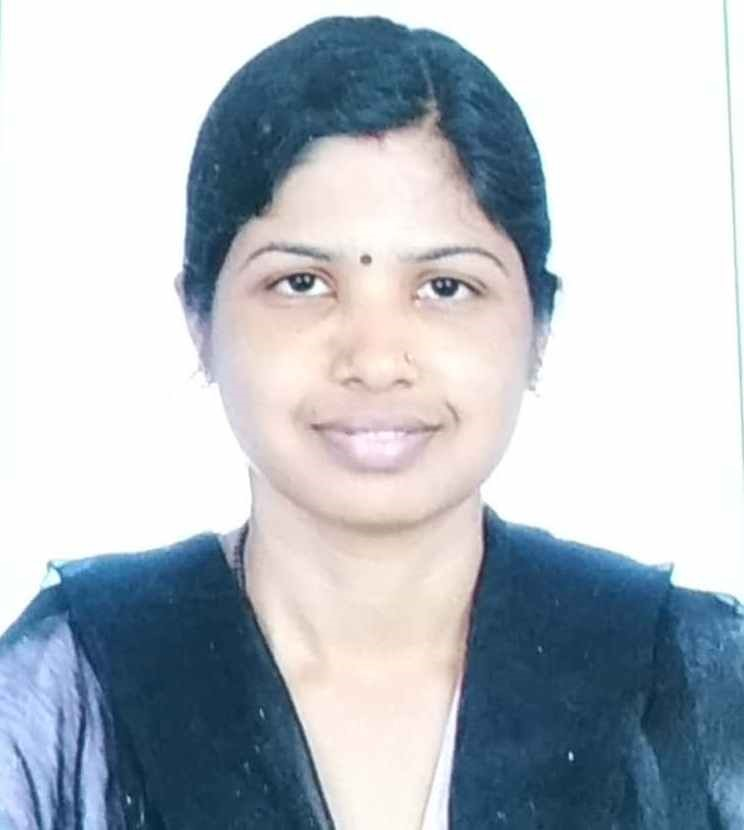### Mrs. Kavitha.B

Assistant Professor

### Mrs. Kavitha.B

Assistant Professor
B.Ed, M.Sc. ,(Ph.D)
08258 - 262724
kavitha.kavipai.pai@gmail.com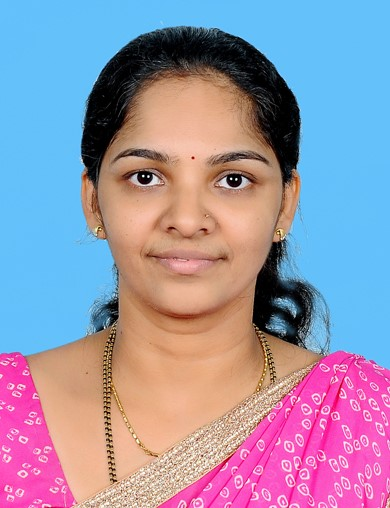### Mrs. Nisha Kumari

Assistant Professor

### Mrs. Nisha Kumari

Assistant Professor
M.Sc. ,B.Ed.
08258 - 262724
nishaprakashputtur@gmail.com### Mrs. Rashmi R

Assistant Professor

### Mrs. Rashmi R

Assistant Professor
M.Sc(Ph.D)
08258 - 262724
rash.sheregar@gmail.com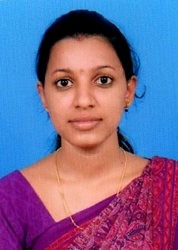### Ms. Sowmya

Assistant Professor

### Ms. Sowmya

Assistant Professor
M.Sc
08258 - 262724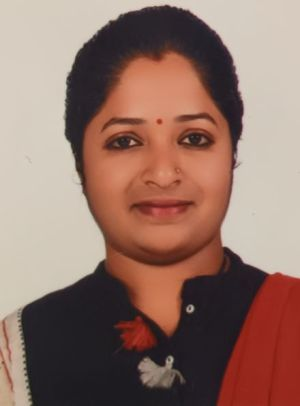Assistant Professor

Assistant Professor
M.Sc., B.Ed
08258-262724